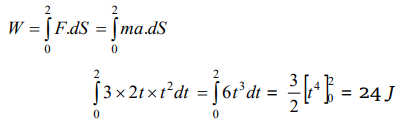## Work, Energy and Power Questions and Answers Part-5

1. Two springs have their force constant as $k_{1}$ and $k_{2}\left(k_{1}>k_{2}\right)$   . When they are stretched by the same force
a) No work is done in case of both the springs
b) Equal work is done in case of both the springs
c) More work is done in case of second spring
d) More work is done in case of first spring

Explanation: $W = \frac{F^{2}}{2k}$
If both springs are stretched by same force2. A spring of force constant 10 N/m has an initial stretch 0.20 m. In changing the stretch to 0.25 m, the increase in potential energy is about
a) 0.1 joule
b) 0.2 joule
c) 0.3 joule
d) 0.5 joule

Explanation:3. The potential energy of a certain spring when stretched through a distance ‘S’ is 10 joule. The amount of work (in joule) that must be done on this spring to stretch it through an additional distance ‘S’ will be
a) 30
b) 40
c) 10
d) 20

Explanation:4. Two springs of spring constants 1500 N/m and 3000 N/m respectively are stretched with the same force. They will have potential energy in the ratio
a) 4 : 1
b) 1 : 4
c) 2 : 1
d) 1 : 2

Explanation:5. A spring 40 mm long is stretched by the application of a force. If 10 N force required to stretch the spring through 1 mm, then work done in stretching the spring through 40 mm is
a) 84 J
b) 68 J
c) 23 J
d) 8 J

Explanation:6. A position dependent force $F=7-2x+3x^{2}$   newton acts on a small body of mass 2 kg and displaces it from $x=0$ to $x=5m$ . The work done in joules is
a) 70
b) 270
c) 35
d) 135

Explanation:7. A body of mass 3 kg is under a force, which causes a displacement in it is given by $S=\frac{t^{3}}{3}$ (in m).Find the work done by the force in first 2 seconds
a) 2 J
b) 3.8 J
c) 5.2 J
d) 24 J

Explanation:8. The force constant of a wire is k and that of another wire is 2k. When both the wires are stretched through same distance, then the work done
a) $W_{2}=2W_1^2$
b) $W_{2}=2W_{1}$
c) $W_{2}=W_{1}$
d) $W_{2}=0.5W_{1}$

Explanation:9. A body of mass 0.1 kg moving with a velocity of 10 m/s hits a spring (fixed at the other end) of force constant 1000 N/m and comes to rest after compressing the spring. The compression of the spring is
a) 0.01m
b) 0.1m
c) 0.2m
d) 0.5m